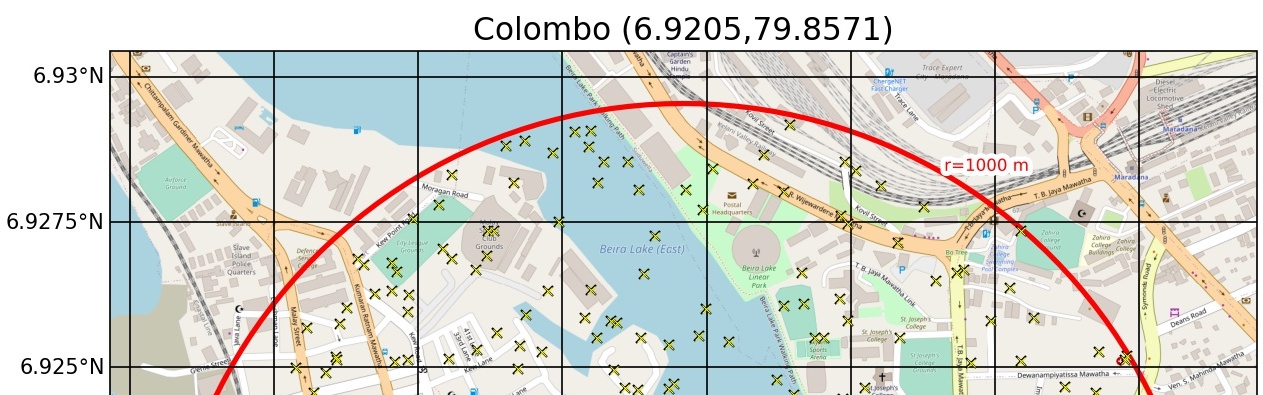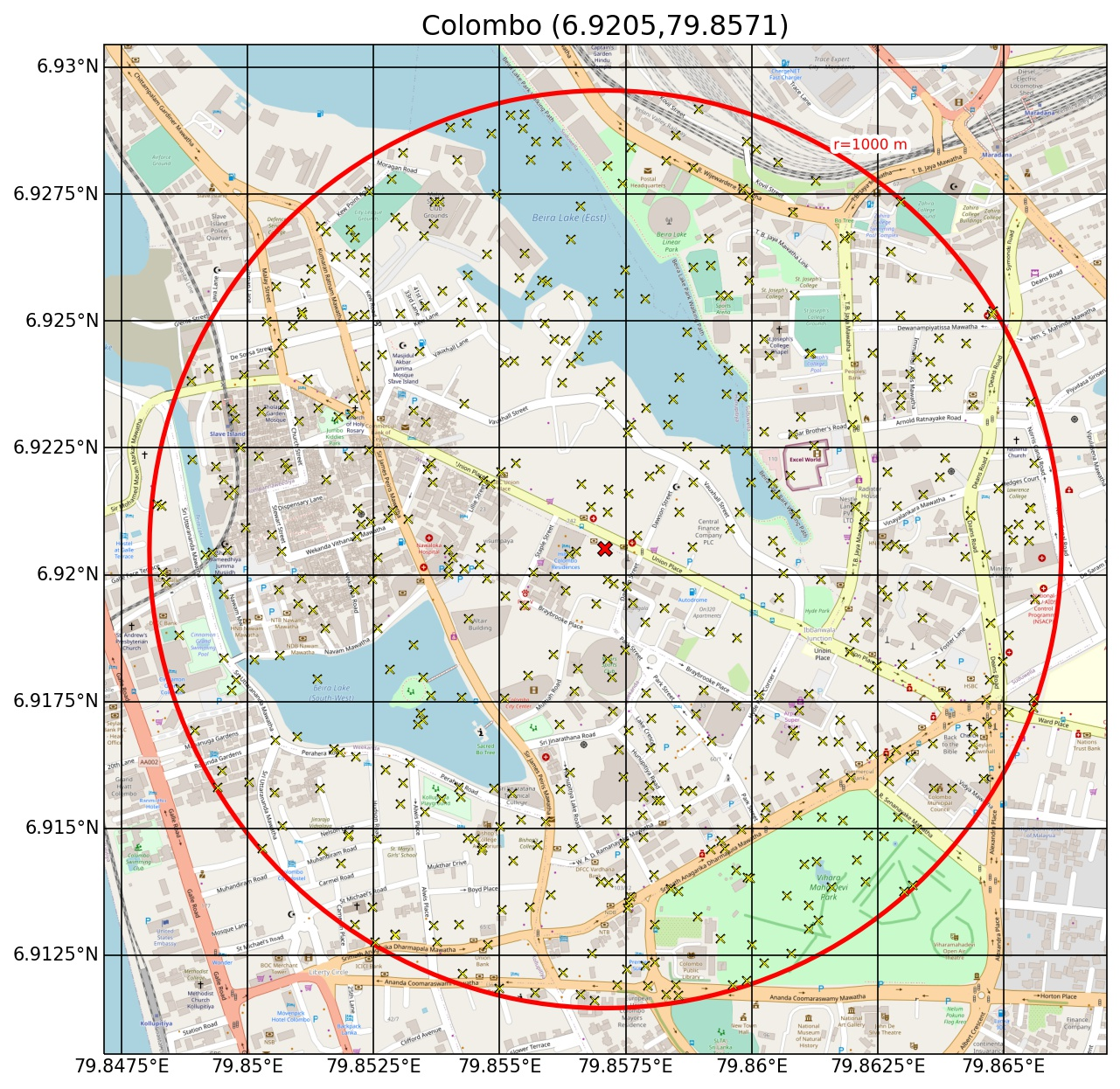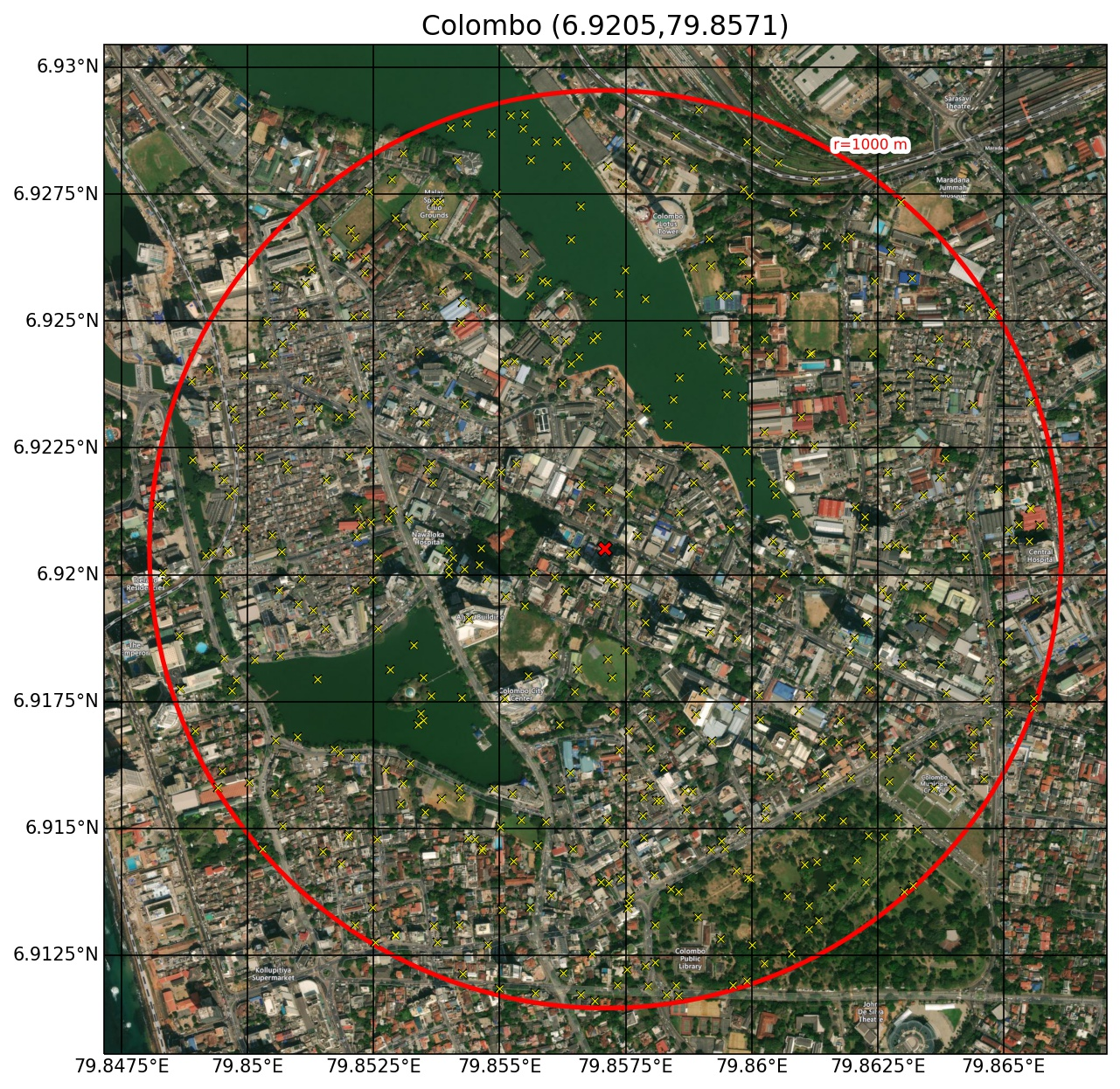# Plotting OpenStreetMap images with Cartopy

This post shows code to import OpenStreetMap and satellite images into Python’s Cartopy.

Inputs are:

• latitude
• longitude
• style (map or satellite)
• npoints (number of random points to plot within the circle)

Note this is only complicated because of the satellite images, for only OpenStreetMap images just follow an example like this.

## Basis

For modelling urban environments I need to know the typical fractions of land use in an area. For this I needed to plot a satellite or map-style image, along with a series of randomly placed markers so I can later count and document the fractions of different areas; buildings, streets, vegetation, water etc.

This turned out to be much more complicated than I first assumed it would be (as seen from the large number of python modules I needed to import). I also borrowed heavily from Joshua Hrisko excellent post on the subject.

But I now have code which will take in any latitude and longitude and produce either satellite or map-style plots as shown below.Images available under the Open Database Licence © OpenStreetMap contributors 2021

## Inputs

The function requires a latitude, longitude and title. Styles, sizes and points are optional inputs.

The circle radius shown here is 1000 m, and always a geodesic distance from the centre point.

The points are randomly and uniformly distributed within the circle, and their locations are reproducable through seeding the random call with a standard integer.

Finally, the scale of the images imported from OpenStreetMap are automatically adapted based on the radius of the circle input, or can be manually chosed integers (1-19).

## Code

The Python code to create these plots is shown below.

__title__   = 'Plot OpenStreetMap site map'
__version__ = 'v1.0 (2021-03-02)'
__author__  = 'Mathew Lipson'
__email__   = 'm.lipson@unsw.edu.au'

import os
import numpy as np
import matplotlib.pyplot as plt
import matplotlib.patches as patches
import matplotlib.patheffects as pe
import cartopy
import cartopy.geodesic as cgeo
import cartopy.crs as ccrs

import cartopy.io.img_tiles as cimgt
import io
from urllib.request import urlopen, Request
from PIL import Image
import shapely

projpath = '.'

##########################################################################

def main():

sitename = 'Colombo'
lat = 6.9205
lon = 79.8571

# style can be 'map' or 'satellite'

for style in ['map','satellite']:

osm_image(lon, lat, sitename=sitename,

return

##########################################################################

'''This function makes OpenStreetMap satellite or map image with circle and random points.
Change np.random.seed() number to produce different (reproducable) random patterns of points.
Also review 'scale' variable'''

if style=='map':
## MAP STYLE
cimgt.OSM.get_image = image_spoof # reformat web request for street map spoofing
elif style =='satellite':
# SATELLITE STYLE
cimgt.QuadtreeTiles.get_image = image_spoof # reformat web request for street map spoofing
else:
print('no valid style')

stroke = [pe.Stroke(linewidth=1, foreground='w'), pe.Normal()]

############################################################################

plt.close('all')
fig = plt.figure(figsize=(10,10)) # open matplotlib figure
ax = plt.axes(projection=img.crs) # project using coordinate reference system (CRS) of street map
data_crs = ccrs.PlateCarree()

ax.set_title(f'{sitename} ({lat},{lon})',fontsize=15)

# auto-calculate scale
scale = (scale<20) and scale or 19

# or change scale manually
# NOTE: scale specifications should be selected based on radius
# but be careful not have both large scale (>16) and large radius (>1000),
#  it is forbidden under [OSM policies](https://operations.osmfoundation.org/policies/tiles/)
# -- 2     = coarse image, select for worldwide or continental scales
# -- 4-6   = medium coarseness, select for countries and larger states
# -- 6-10  = medium fineness, select for smaller states, regions, and cities
# -- 10-12 = fine image, select for city boundaries and zip codes
# -- 14+   = extremely fine image, select for roads, blocks, buildings

ax.set_extent(extent) # set extents

ax.plot(lon,lat, color='black', marker='x', ms=7, mew=3, transform=data_crs)
ax.plot(lon,lat, color='red', marker='x', ms=6, mew=2, transform=data_crs)

if npoints>0:
# set random azimuth angles (seed for reproducablity)
np.random.seed(1235)
rand_azimuths_deg = np.random.random(npoints)*360

# set random distances (seed for reproducablity)
np.random.seed(6341)

rand_lon = cgeo.Geodesic().direct((lon,lat),rand_azimuths_deg,rand_distances)[:,0]
rand_lat = cgeo.Geodesic().direct((lon,lat),rand_azimuths_deg,rand_distances)[:,1]

ax.plot(rand_lon,rand_lat,color='black',lw=0,marker='x',ms=4.5,mew=1.0,transform=data_crs)
ax.plot(rand_lon,rand_lat,color='yellow',lw=0,marker='x',ms=4,mew=0.5,transform=data_crs)

geom = shapely.geometry.Polygon(circle_points)

stroke = [pe.Stroke(linewidth=5, foreground='w'), pe.Normal()]
fontsize=8, ha='left',va='bottom', path_effects=stroke, transform=data_crs)

gl = ax.gridlines(draw_labels=True, crs=data_crs,
color='k',lw=0.5)

gl.top_labels = False
gl.right_labels = False
gl.xformatter = cartopy.mpl.gridliner.LONGITUDE_FORMATTER
gl.yformatter = cartopy.mpl.gridliner.LATITUDE_FORMATTER

# plt.show()

return

def calc_extent(lon,lat,dist):
'''This function calculates extent of map
Inputs:
lat,lon: location in degrees
dist: dist to edge from centre
'''

dist_cnr = np.sqrt(2*dist**2)
top_left = cgeo.Geodesic().direct(points=(lon,lat),azimuths=-45,distances=dist_cnr)[:,0:2]
bot_right = cgeo.Geodesic().direct(points=(lon,lat),azimuths=135,distances=dist_cnr)[:,0:2]

extent = [top_left, bot_right, bot_right, top_left]

return extent

def image_spoof(self, tile):
'''this function reformats web requests from OSM for cartopy
Heavily based on code by Joshua Hrisko at:
https://makersportal.com/blog/2020/4/24/geographic-visualizations-in-python-with-cartopy'''

url = self._image_url(tile)                # get the url of the street map API
req = Request(url)                         # start request
fh = urlopen(req)
im_data = io.BytesIO(fh.read())            # get image
fh.close()                                 # close url
img = Image.open(im_data)                  # open image with PIL
img = img.convert(self.desired_tile_form)  # set image format
return img, self.tileextent(tile), 'lower' # reformat for cartopy

if __name__ == "__main__":

main()